# Solving Pythagorean Triplets and Special Triangles in GMAT

Let us try to tackle the problem of Special Right Triangles and Pythagorean Triplets, today. The theory will be followed by suitable examples for special right triangles. As you must be aware, the Pythagorean Theorem is one of the most used tools in geometry.  Its three main features are:

1) One of the angles is a right angle

2) The sides which are adjacent to the right angle are termed as the base and perpendicular/height

3) The third side is known as the hypotenuse

For the figure given below, the hypotenuse is ‘c’, the base is ‘b’ and the perpendicular or height is ‘a’.  As proposed by Pythagoras, the sum of square of sides adjacent to right angle is equal to the square of the third side, better known as the hypotenuse. Putting these words into equation form, we see:

a^2+ b^2=c^2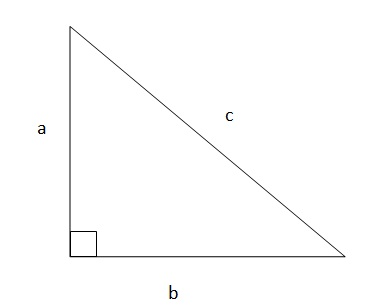Two major takeaways from this theorem are:

a) It is applicable only to right angled triangles
b) The choice of base and perpendicular is irrelevant as far as the two sides adjacent to the right angled triangle are named.

Let us quickly see one application of Pythagorean Theorem before moving on to Special Right Triangles.

Example: John wants to visit his friend Ron’s house. He starts from his house and travels 3 km south before reaching a traffic signal. From there, he takes a 90 degree turn left and travels 4 km to reach Ron’s house. So how far is Ron’s house from John’s house?

Let’s approach this question step by step

Step 1: Make a pictorial representation of the problem. Assume that John’s house is point A, the traffic signal is B, and Ron’s house is C.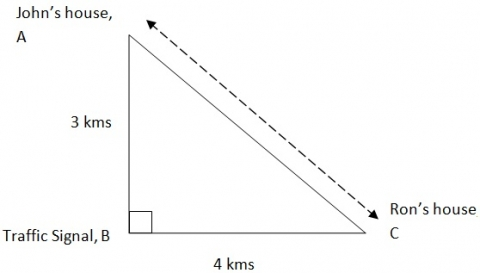Step 2: Mark the distances as shown. John’s house at point A to the Traffic Signal at B is 3 km, and from the Traffic Signal at B to Ron’s house at C is 4 km.

Step 3: The problem is to find the distance between Ron’s house and John’s house. As seen clearly from the pictorial representation, this can be solved by applying Pythagorean Theorem.

Step 4: Solving using the Pythagorean Theorem

AB^2+BC^2=AC^2

So, AC= sqrt(AB^2+BC^2)

AC= sqrt (3^2+4^2)

Therefore, distance  AC= sqrt(25) = 5

Thus, the solution to the problem is: John’s house is 5 km from Ron’s house.

Pythagorean Triplets

One concept we can consider before moving forward is ‘Pythagorean Triplets’. As is clear from the name, these are sets of numbers that follow Pythagorean Theorem. Some examples are 3, 4, 5;

5, 12, 13;

8, 15, 17 and so on.

Quick tips

1) Knowledge of Pythagorean triplets in advance makes it easier to identify the number series and saves valuable time during exams.

2) If you multiply the triplet with any common number, the new triplet will also follow the Pythagorean rule.

Example 3, 4, 5 multiplied by two gives 6, 8, 10, which is also a Pythagorean triplet.

3) There are 16 such triplets under 100. I have given three examples above; try to learn the other 13 pairs too.

There are multiple ways in which Pythagorean Theorem can be applied, but some of the common applications in geometry are:

1) Using the standard form of the theorem to calculate distances and heights. This also has a good deal of applications in trigonometry.

2) The converse of theorem (i.e. if the sum of the square of two sides is equal to square of the third side, then it is a right angled triangle) also has a good variety of applications, especially in solving problems in geometry.

Special Right Triangles

Now let’s turn our attention to Special Right Triangles.

The first thing that comes to mind is: what is special about these triangles? The Special feature lies in the relationship of the sides or the angles. Let’s examine them one by one.

a) Side-based Special triangles

The special feature of side-based triangles comes from a ratio formation between the three sides. Pythagorean triplet is one such formulation.

3:4:5 ratio; 9:40:41 ratio

For example, suppose we have a problem like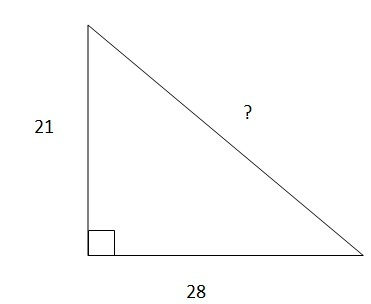The obvious way is to apply the Pythagoras theorem and calculate the third side, but there is a simpler way. On closer observation we can see that 21:28:?  follows the 3:4:5 pattern with the original triplet multiplied by 7. Thus, the intended solution is 7*5=35

Hence  knowledge of special triangle feature helps us to save valuable time by speeding up the pace of calculations.

The same property can be observed in the case of 9:40:41 and other similar triplets.

b) Angle-based special triangles

Two of the most useful cases are the 45:45:90 triangle and 30:60:90 triangle. In the case of the 45:45:90 triangle, the sides of the triangle would be in the ratio of n:n:n sqrt(2)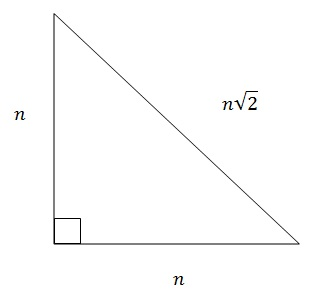For example, if we have a problem to find out the length of the hypotenuse when the angles are 45:45:90 and the length of one side is 5 cm, then the length of the hypotenuse will be 5
xsqrt(2) cm.

Quick tip: just a single bit of information (i.e. one of the angles of a right angled triangle is 45°) should be enough to tell that it is a 45:45:90 special triangle. This would help minimize the time spent calculating the lengths of the sides.

Case of 30:60:90 special triangle: these sides will follow the ratio of n:n
xsqrt(3):2n for the two sides and the hypotenuse respectively.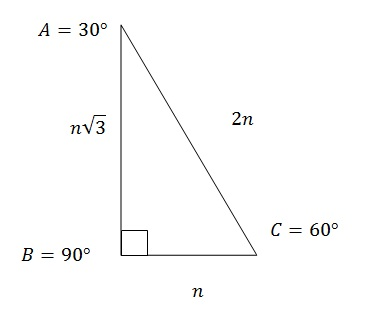Quick tip: If one of the angles of a right angled triangle is given as 30° or 60°, we can easily figure out the property that the sides of this triangle will follow the ratio of n:n
xsqrt(3):2n

Importance of Pythagorean Theorem and Special Triangles

1) Lessens the pressure on students if they know the short cuts and properties of special triangles

2) Reduces the time taken to calculate complex triplets

3) Memorizing Pythagorean triplets under 100 is helpful in the exam.

Author :GoGMAT, founded in 2009, is an adaptive GMAT preparation platform developed by the best instructors in the industry (with 740+ GMAT scores and strong teaching experience). GoGMAT team consists of high profile members, successful entrepreneurs, experts in educational field, top-notch business school alumni with successful track records as investment bankers and management consultants. Our GMAT experts, university professors and successful entrepreneurs, analyzed GMAT test algorithms for almost 12 months.  We used their knowledge and skills as the basis to create one of the most efficient solutions in the world for GMAT preparation.

Disclaimer: The articles provided by GoGMAT are for informational purposes only and cannot be copied or redistributed without the written permission by GoGMAT. F1GMAT publishes guest posts by authors from all over the world. We do not accept any responsibility or liability for the information contained in the article or claims of copyright violation. However, we take immediate action when any copyright violations are reported. Report copyright violation1. Complete GMAT RC Questions in less than 1 minute and 50 seconds
3. Take Notes Effectively
4. Collect and Interpret Facts
5. Speed up Summary Creation
6. Remember Information
7. Question the Author

12. Learn to Answer GMAT organization of passage Question
13. Learn to identify the style/tone or attitude of the author

Mastering GMAT Critical ReasoningAfter you read F1GMAT’s Mastering GMAT Critical Reasoning Guide, you will learn:

How to overcome flawed thinking in GMAT Critical Reasoning?

How to spot Inconsistencies in Arguments

How to eliminate out of scope answer choices using Necessary and Sufficient Conditions

How to Paraphrase GMAT Critical Reasoning Question

How to Answer Assumption Question Type

How to Answer Conclusion Question Type

How to Answer Inference Question Type

How to Answer Strengthen Question Type

How to Answer Weaken Question Type

How to Answer bold-faced and Summary Question Types

How to Answer Parallel Reasoning Questions

How to Answer the Fill in the Blanks Question

Get F1GMAT's Newsletters (Best in the Industry)

• Ranking Analysis
• Post-MBA Salary Trends
• Post-MBA Job Function & Industry Analysis
• Post-MBA City Review
• MBA Application Essay Tips
• School Specific Essay Tips
• GMAT Preparation Tips